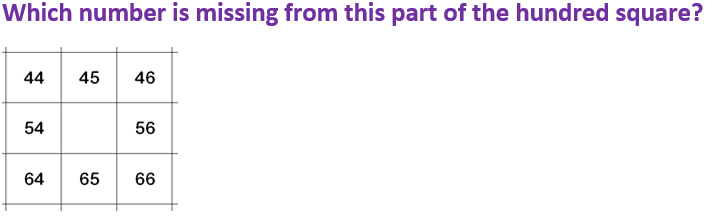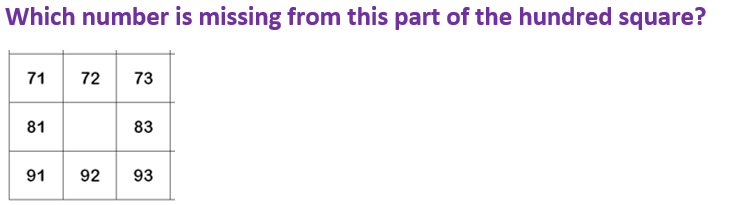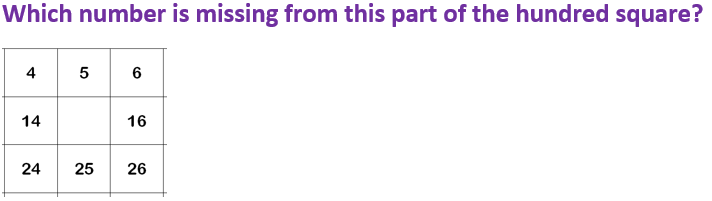# KS1 Maths Quiz - Year 2 Numbers - Place Value Problem Solving (Questions)

This quiz addresses the requirements of the National Curriculum KS1 Maths and Numeracy for children aged 6 and 7 in year 2. Specifically this quiz is aimed at the section dealing with using place value and number facts to solve problems.

Children in Year 2 begin to learn about what each digit in a number represents, or its place value. For example, in the number 34, there are 3 tens and 4 units. They can use this knowledge of place values to solve problems using 100 squares, answer more than/less than questions, solve money problems and also use it to answer number bond questions.

1.[ ] 55 [ ] 85 [ ] 59 [ ] 32
2.[ ] 85 [ ] 82 [ ] 38 [ ] 88
3.[ ] 6 [ ] 15 [ ] 23 [ ] 27
4. Which number is 10 more than 43?
 [ ] 44 [ ] 54 [ ] 63 [ ] 53
5. Which number is 4 more than 65?
 [ ] 66 [ ] 69 [ ] 79 [ ] 80
6. Which number is 20 less than 80?
 [ ] 60 [ ] 67 [ ] 63 [ ] 70
7. Kate has 42p. Joe has 10p more. How much does Joe have?
 [ ] 62p [ ] 52p [ ] 32p [ ] 22p
8. I have £1 and spend 50p. How much do I have left?
 [ ] 80p [ ] 20p [ ] 60p [ ] 50p
9. Which number is missing from this number sentence?
12 + ? = 20
 [ ] 8 [ ] 18 [ ] 12 [ ] 10
10. Which number is missing from this number sentence?
20 - ? = 13
 [ ] 17 [ ] 23 [ ] 7 [ ] 13KS1 Maths Quiz - Year 2 Numbers - Place Value Problem Solving (Answers)
1.[x] 55 [ ] 85 [ ] 59 [ ] 32
55 is one more than 54, and 10 more than 45
2.[ ] 85 [x] 82 [ ] 38 [ ] 88
82 is one less than 83, and ten less than 92
3.[ ] 6 [x] 15 [ ] 23 [ ] 27
15 is one less than 16 and ten more than 5
4. Which number is 10 more than 43?
 [ ] 44 [ ] 54 [ ] 63 [x] 53
‘10 more’ means adding 10 to the number
5. Which number is 4 more than 65?
 [ ] 66 [x] 69 [ ] 79 [ ] 80
‘4 more’ means adding 4 to 65
6. Which number is 20 less than 80?
 [x] 60 [ ] 67 [ ] 63 [ ] 70
’20 less’ means counting back 20 from 80, or subtracting 20
7. Kate has 42p. Joe has 10p more. How much does Joe have?
 [ ] 62p [x] 52p [ ] 32p [ ] 22p
10p more means adding one 10 in the tens column
8. I have £1 and spend 50p. How much do I have left?
 [ ] 80p [ ] 20p [ ] 60p [x] 50p
£1 is 100p. 50p less than this is 50p
9. Which number is missing from this number sentence?
12 + ? = 20
 [x] 8 [ ] 18 [ ] 12 [ ] 10
Knowing numbers bonds or pairs to 20 helps here
10. Which number is missing from this number sentence?
20 - ? = 13
 [ ] 17 [ ] 23 [x] 7 [ ] 13
13 + 7 = 20, so 20 – 7 must be 13!